# AP Board 7th Class Maths Solutions Chapter 10 Construction of Triangles InText Questions

SCERT AP 7th Class Maths Solutions Pdf Chapter 10 Construction of Triangles InText Questions and Answers.

## AP State Syllabus 7th Class Maths Solutions 10th Lesson Construction of Triangles InText Questions

[Page No. 67]

Look at the picture and answer the following questions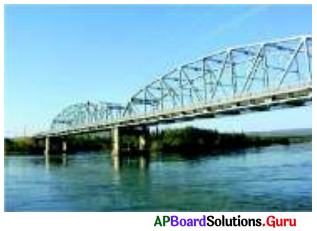Question 1.
Name some things from your daily life that look like triangles.
Samosa, chapathi, window elevations, house tops, bridge trusses, floor tessellations.

Question 2.
What are the types of triangles can see in the picture?
Right triangles,
Equilateral triangles.

Question 3.
Do you think all triangles shown are similar with their properties? What are they?
Yes. All right triangles are similar.

All triangles are similar because they have
(a) right angle
(b) equal side and
(c) equal hypotenuse.Check Your Progress [Page No. 69]

Question 1.
Construct an equilateral triangle ∆XYZ with XY = 4 cm.
Given sides of an equilateral triangle ∆XYZ is XY = 4 cm.
In equilateral triangle all sides ,gresequal in length
∴ XY = YZ = ZX = 4 cm.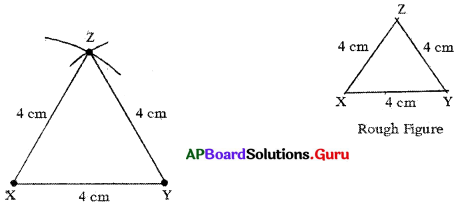Steps of Construction:

1. Draw a rough sketch of the triangle and label it with the given measurements.
2. Draw a line segment with XY = 4 cm,
3. Draw an arc with centre X and radius 4 cm.
4. Draw another arc with centre Y and radius 4 cm to intersecting the previous arc at Z.
5. Join XZ andYZ.

Hence, required ∆XYZ is constructed with the given measurements.

Question 2.
Construct an isosceles triangle ∆PQR With PQ = PR = 3 cm, QR = 5 cm.
Given sides of an isosceles triangle ∆PQR are PQ = PR = 3 cm and QR = 5 cm
In isosceles triangle two sides are equal.
∴ PQ = PR = 3 cm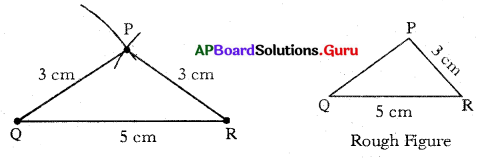Steps of construction:

1. Draw a rough sketch of the triangle and label it with the given measurements.
2. Draw a line segment QR = 5 cm.
3. Draw an arc with centre Q and radius 3 cm.
4. Draw another arc with centre R and same radius (3 cm) to intersect the previous arc at P.
5. Join PQ and PR.

Hence, required APQR is constructed with the given measurements.

Let’s Think [Page No. 69]

Can you construct ∆ABC with AB = 4 cm, BC = 5 cm and CA =10 cm? Why? Justify your answer.
Given sides of a ∆ABC are AB = 4 cm, BC = 5 cm and CA =10 cm.
In any triangle sum of any two sides is always greater than the third side.
AB + BC = 4 cm + 5 cm = 9 cm <10 cm.
Sum of AB + BC < AC
So, with the given measurements construction of ∆ABC is not possible.

Check Your Progress [Page No. 72]

Question 1.
Construct ∆MAT with measurements MA = 5.5 cm, MT = 4 cm and ∠M=70°.
Given measurements of ∆MAT are MA = 5.5 cm, MT = 4 cm and ∠M = 70°.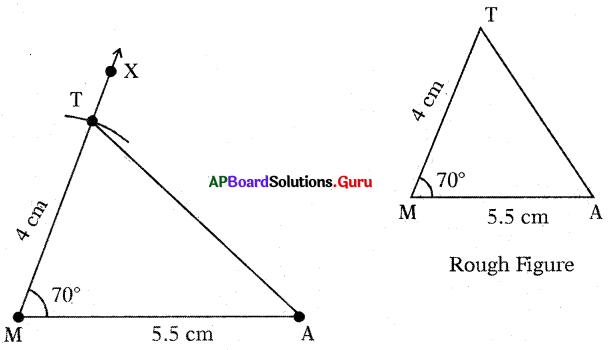Steps of Construction:

1. Draw a rough sketch of the triangle and label it with the given measurements.
2. Draw a line segment with MA = 5.5 cm.
3. Draw a ray $$\overrightarrow{\mathrm{MX}}$$ such that ∠AMX = 70°
4. Draw an arc with centre M and radius 4 cm, to intersect the $$\overrightarrow{\mathrm{MX}}$$ at point T.
5. Join AT.

Hence, required ∆MAT is constructed with the given measurements.Let’s Explore [Page No. 72]

Question 1.
Construct the triangle with the measurements of AB = 7cm, ∠B = 60° and ∠C = 70°.
Given measurements of ∆ABC are AB = 7 cm, ∠B = 60° and ∠C = 70°.
In ∆ABC, ∠A + ∠B + ∠C = 180°
∠A + 60° + 70° = 180° .
∠A + 130° = 180°
∠A = 180° – 130° = 50°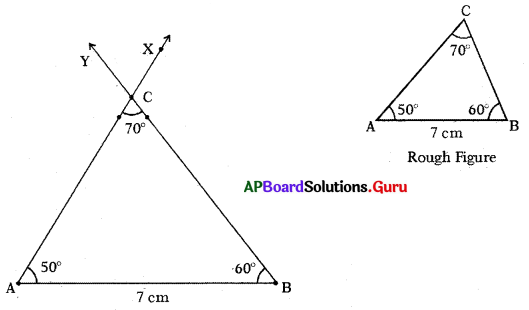Steps of Construction:

1. Draw a rough sketch of triangle and label it with given measurements.
2. Draw a line segment with AB = 7 cm.
3. Draw a ray BY such that ABY = 60°.
4. Draw another ray AX such that BAX = 50°.
5. Name the intersecting of rays AX and BY is C.

Hence, required ∆ABC is constructed with the given measurements.

Check Your Progress [Page No. 73]

Question 1.
Construct ∆ABC with the measurements ∠A = 90°, ∠C = 50° and AC = 8 cm.
Given measurements of ∆ABC are ∠A = 90°, ∠C = 50° and AC = 8 cm.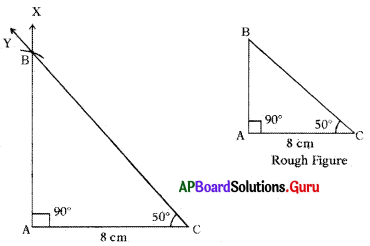Steps of Construction:

1. Draw a rough sketch of triangle and label it with given measurements.
2. Draw a line segment with AC = 8 cm.
3. Draw a ray AX such that ∠CAX = 90°.
4. Draw another ray CY such that ∠ACY = 50°.
5. Name the intersecting point of AX and CY as B.

Thus, required ∆ABC is constructed with the given measurements.

Let’s Think [Page No. 73]

Construct a triangle with angles 100°, 95° and a side of length of your choice. Can1 you construct the triangle?
No. It is not possible. We know that the sum of the angles of a triangle is 180°.
But, given two angles are 100° and 95°. Their sum is greater than 180°.
So, we cannot construct the triangle.

Examples:

Question 1.
Construct ∆ABC with slides AB = 6 cm, BC = 4 cm and AC = 5 cm.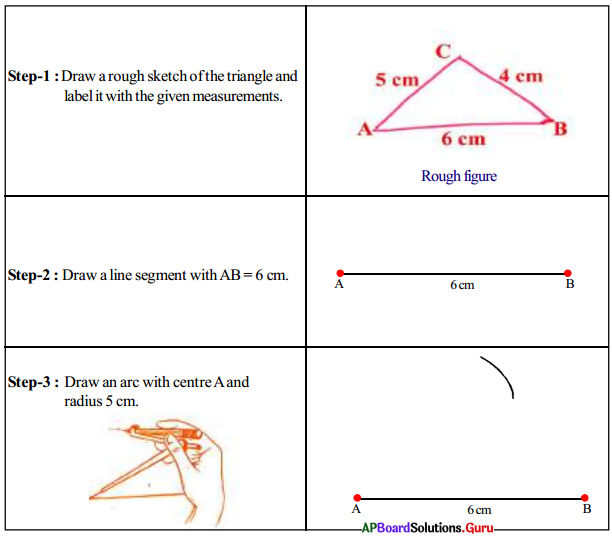Question 2.
Construct ∆EFD with the measurements ∠F = 70°, EF = 4 cm and FD = 5 cm.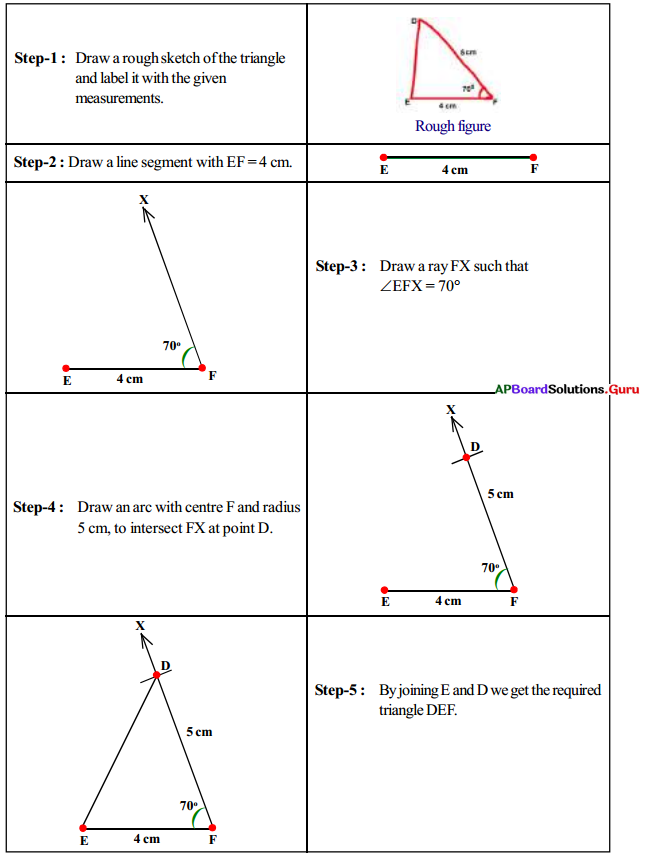Question 3.
Construct ∆ABC with AB = 6 cm, BC = 7 cm, ∠BAC = 80°.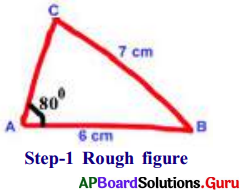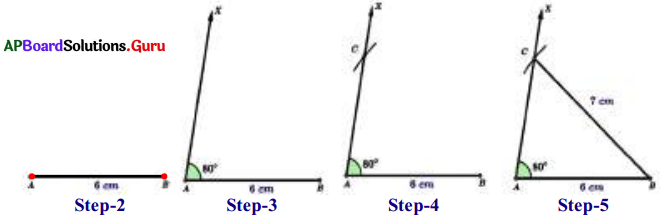• Step-1: Draw a rough sketch of ∆ABC and label it with the given measurements.
• Step-2: Draw a line segment AB of length 6 cm.
• Step-3: Draw a ray AX such that ∠BAX = 80°.
• Step-4: Draw an arc with center B and radius 7 cm to Intersect the ray AX at C.
• Step-5: Join B and C to get the required ∆ABC.

Question 4.
Construct a right angled triangle ∆XYZ, XY = 4 cm, XZ = 6.5 cm and ∠Y = 90°.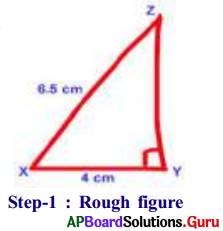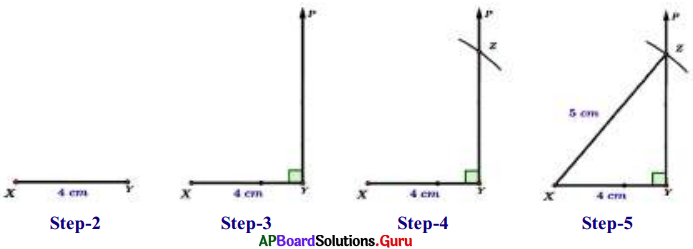• Step-1: Draw a rough sketch of ∆XYZ and label it with given measurements.
• Step-2: Draw a line segment with XY = 4 cm.
• Step-3: Draw a ray YP such that ∠XYP = 90°.
• Step-4: Draw an arc with center X and radius 6.5 cm to intersect the Ray YP at Z.
• Step-5: Join X and Z to get the required ∆XYZ.

Question 5.
Construct ∆PEN with measurements PE. = 7 cm, ∠PEN = 25° and ∠EPN = 60°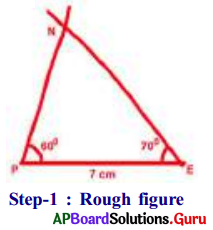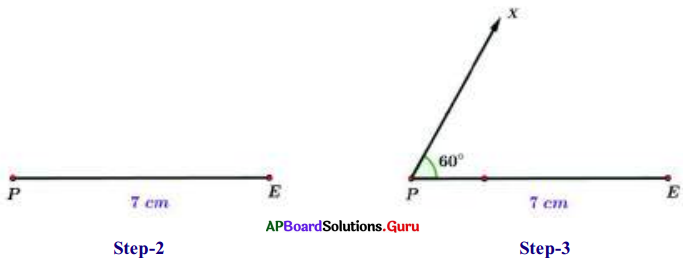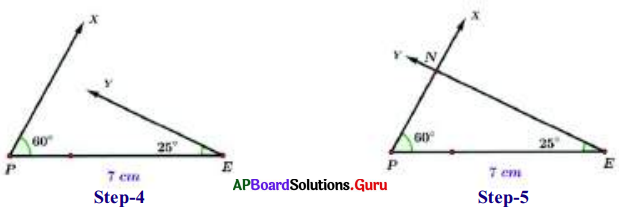• Step-1: Draw a rough sketch of the triangle and label it with given measurements.
• Step-2: Draw a line segment with PE = 7 cm,
• Step-3: Draw a ray PX such that ∠EPX = 60°.
• Step-4: Draw another ray EY such that ∠PEY = 25°.
• Step-5: Rays PX and EY intersect each other at N.

Hence, we get the required ∆PEN.
(If necessary, extend the rays to form a triangle.)

Question 6.
Construct ∆MPC the triangle with the measurements MP = 4 cm, ∠P = 45° arid ∠C = 80°.
Now we have to find out the third angle.
We know that the sum of three angles in a triangle is 1800.
So, ∠M + ∠P + ∠C = 180°
⇒ ∠M + 450 + 80° = 180°
⇒ ∠M + 125° = 180°
⇒ ∠M = 180 – 125 = 55°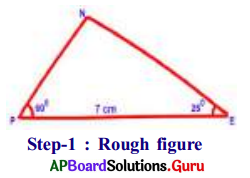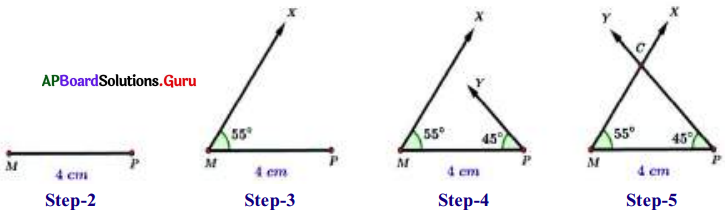• Step-1: Draw a rough sketch of the triangle and label it with given measurements.
• Step-2: Draw a line segment with MP = 4 cm.
• Step-3: Draw a ray MX such that ∠PMX = 55°.
• Step-4: Draw another ray PY such that ∠MPY = 45°.
• Step-5: Rays MX and PY intersect each other at C. (If necessary extend the rays to ‘ form a triangle.)

Hence, we get the required ∆MPC.
Verification: By using the protractor and check whether ∠C is equal to 80° or not.Practice Questions [Page No. 78]

Find the number of triangles in the given figures.

Question 1.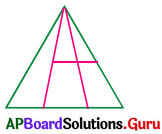(a) 8
(b) 9
(c) 10
(d) 12
(b) 9

Explanation: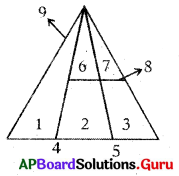Question 2.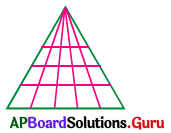(a) 60
(b) 65
(c) 84
(d) 90
(a) 60

Explanation: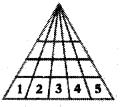Number of rows = 4
Sum of numbers in each row = 1 + 2 + 3 + 4 + 5 = 15
Number of triangles in the picture = 4 × 15 = 60

Question 3.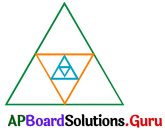(a) 12
(b) 13
(c) 14
(d) 15
(b) 13

Explanation:
Formula to count number of triangles = 4n + 1
Here n = number of embedded triangles in outer triangle
In the figure n = 3
So, number of triangles = 4(3) + 1 = 12 + 1 = 13

Question 4.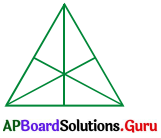(a) 16
(b) 13
(c) 9
(d) 7
(a) 16

Question 5.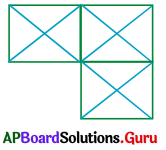(a) 21
(b) 23
(c) 25
(d) 29
(d) 29

Explanation: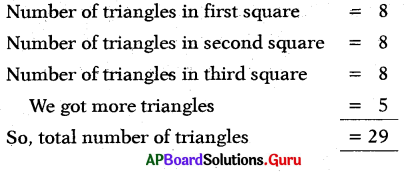Question 6.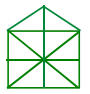(a) 10
(b) 19
(c) 21
(d) 23
(c) 21

Explanation: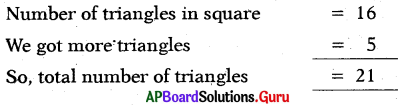Question 7.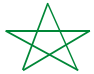(a) 5
(b) 6
(c) 8
(d) 10
(c) 8Question 8.(a) 9
(b) 10
(c) 11
(d) 12
(a) 9

Explanation: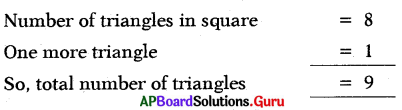Question 9.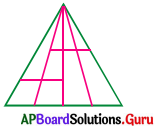(a) 19
(b) 20
(c) 16
(d) 14
(a) 19

Question 10.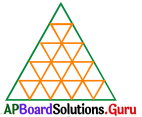(a) 56
(b) 48
(c) 32
(d) 60
Formula to count number of triangles = $$\frac{\mathrm{n}(\mathrm{n}+2)(2 \mathrm{n}+1)}{8}$$
Number of triangles = $$\frac{5(5+2)(2 \times 5+1)}{8}=\frac{5 \times 7 \times 11}{8}$$ = 48.1